# Integration by long division

Since integration is the inverse of differentiation, you can think of integration as “dividing” by d.

J. P. Ballantine  shows that you can formally divide by d and get the correct integral. For example, he arrives atusing long division!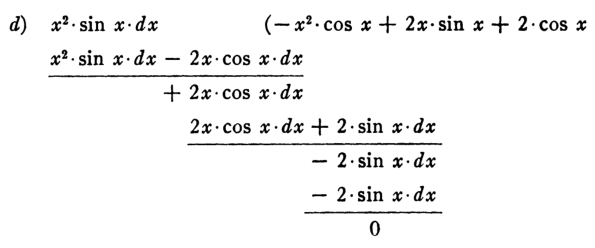J. P. Ballantine. Integration by Long Division. The American Mathematical Monthly, Vol. 58, No. 2 (Feb., 1951), pp. 104-105

# Currying in calculus, PDEs, programming, and categoriesCurrying is a simple but useful idea. (It’s named after logician Haskell Curry  and has nothing to do with spicy cuisine.) If you have a function of two variables, you can think of it as a function of one variable that returns a function of one variable. So starting with a function f(x, y), we can think of this as a function that takes a number x and returns a function f(x, -) of y. The dash is a placeholder as in this recent post.

## Calculus: Fubini’s theorem

If you’ve taken calculus then you saw this in the context of Fubini’s theorem:To integrate the function of two variables f(x, y), you can temporarily fix y and integrate the remaining function of x. This gives you a number, the value of an integral, for each y, so it’s a function of y. Integrate that function, and you have the value of the original function of two variables.

The first time you see this you may think it’s a definition, but it’s not. You can define the integral on the left directly, and it will equal the result of the two nested integrations on the right. Or at least the two sides will often be equal. The conditions on Fubini’s theorem tell you exactly when the two sides are equal.

## PDEs: Evolution equations

A more sophisticated version of the same trick occurs in partial differential equations. If you have an evolution equation, a PDE for a function on one time variable and several space variables, you can think of it as an ODE via currying. For each time value t, you get a function of the spatial variables. So you can think of your solution as a path in a space of functions. The spatial derivatives specify an operator on that space of functions.

(I’m glossing over details here because spelling everything out would take a lot of writing, and might obscure the big idea, which relevant for this post. If you’d like the full story, you can see, for example, my graduate advisor’s book. It was out of print when I studied it, but now it’s a cheap Dover paperback.)

In the Haskell programming language (also named after Haskell Curry) you get currying for free. In fact, there’s no other way to express a function of two variables. For example, suppose you want to implement the function f(xy) = x² + y.

    Prelude> f x y = x**2 + y

Then Haskell thinks of this as a function of one variable (i.e. x), that returns a function of one variable (i.e. f(x, -)) which itself returns a number (i.e. f(x, y)). You can see this by asking the REPL for the type of f:

    Prelude> :info f
f :: Floating a => a -> a -> a


Technically, Haskell, just like lambda calculus, only has functions of one variable. You could create a product datatype consisting of a pair of variables and have your function take that as an argument, but it’s still a function on one variable, though that variable is not atomic.

## Category theory

The way you’d formalize currying in category theory is to say that the following is a natural isomorphism:For more on what Hom means, see this post.

## Related posts

 In concordance with Stigler’s law of eponymy, currying was not introduced by Curry but Gottlob Frege. It was then developed by Moses Schönfinkel and developed further by Haskell Curry.

# Quantifying normal approximation accuracy

Probability is full of theorems that say that probability density approximates another as some parameter becomes large. All the dashed lines in the diagram below indicate a relationship like this.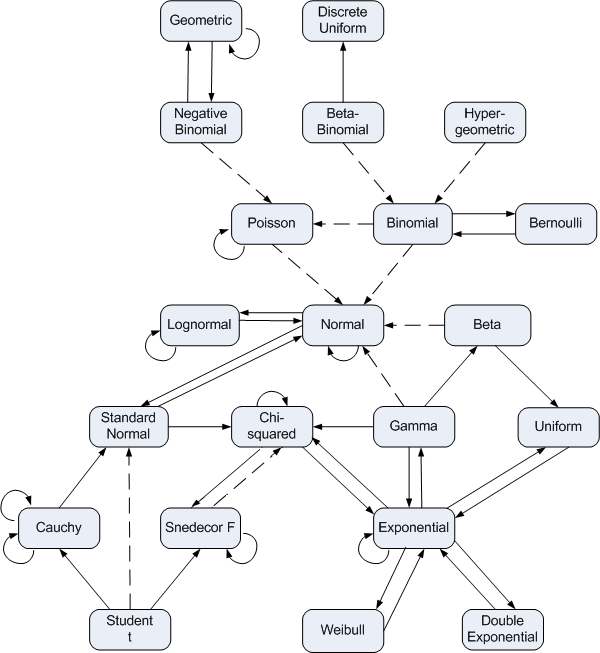You can find details of what everything in the diagram means here.

How can you quantify these approximations? One way is to use Kullback-Leibler divergence.  In this post I’ll illustrate this for the normal approximation to the beta and gamma distributions.

The Kullback-Leibler divergence between two random variables X and Y is defined asWe will compute this integral numerically in the code below to create graphs of how K-L divergence varies with parameters.

Here are the imports we’ll need.

    from scipy.integrate import quad
from scipy.stats import beta, gamma, norm
from scipy import inf
import matplotlib.pyplot as plt


## Beta distribution

As the shape parameters of a beta distribution become large, the probability distribution becomes approximately normal (Gaussian).

Here is code that will take the two shape parameters of a beta distribution, construct a normal approximation by moment matching, and compute the quality of the approximation as measured by Kullback-Leibler divergence.

    def KL_beta_norm(a, b):
b = beta(a, b)
n = norm(b.mean(), b.std())
f = lambda x: -b.pdf(x)*(n.logpdf(x) - b.logpdf(x))
integral, error = quad(f, 0, 1)
return integral


And here we make our plot.

    x = [2**i for i in range(11)]
y = [KL_beta_norm(t, 2*t) for t in x]
plt.plot(x, y)
plt.xscale("log")
plt.yscale("log")
plt.xlabel("a")
plt.ylabel("KL divergence")
plt.title("Comparing beta(a, 2a) and its normal approximation")
plt.savefig("beta_KL.svg")
plt.close()


The result is nearly linear on a log-log scale.I made the b parameter twice the a parameter to show that you don’t need symmetry. When you do have symmetry, i.e a = b, the approximation quality is better and the graph is even straighter.

## Gamma distribution

As the shape parameter of a gamma distribution increases, the probability density becomes more and more like that of a normal distribution. We can quantify this with the following code.

    def KL_gamma_norm(shape):
g = gamma(shape)
n = norm(g.mean(), g.std())
f = lambda x: -g.pdf(x)*(n.logpdf(x) - g.logpdf(x))
mode = max(0, shape-1)
integral1, error1 = quad(f, 0, mode)
integral2, error2 = quad(f, mode, inf)
return integral1 + integral2


The integration code is a little more complicated this time. For small shape parameters, code analogous to that for the beta distribution will work just fine. But for larger parameters, the integration fails. The numerical integration routine needs a little help. The largest contribution to the integral is located near the mode of the gamma distribution. For large shape parameters, the integration routine misses this peak and grossly underestimates the integral. We break the integral into two pieces at the mode of the gamma distribution so the integration routine can’t miss it. This is a small example of why numerical integration cannot be completely automated. You have to know something about what you’re integrating.

(The quad() function has a parameter points to let you tell it about points of interest like this, but it doesn’t work when one of the limits of integration is infinite.)

The plotting code is essentially the same as that for the beta distribution. As before, the plot is linear on a log-log scale.You could do a similar analysis on the other approximations in the distribution relationship diagram above.

# Scaling and Monte Carlo integration methods

Here’s an apparent paradox. You’ll hear that Monte Carlo methods are independent of dimension, and that they scale poorly with dimension. How can both statements be true?

The most obvious way to compute multiple integrals is to use product methods, analogous to the way you learn to compute multiple integrals by hand. Unfortunately the amount of work necessary with this approach grows exponentially with the number of variables, and so the approach is impractical beyond modest dimensions.

The idea behind Monte Carlo integration, or integration by darts, is to estimate the area under a (high dimensional) surface by taking random points and counting how many fall below the surface. More details here. The error goes down like the reciprocal of the square root of the number of points. The error estimate has nothing to do with the dimension per se. In that sense Monte Carlo integration is indeed independent of dimension, and for that reason is often used for numerical integration in dimensions too high to use product methods.

Suppose you’re trying to estimate what portion of a (high dimensional) box some region takes up, and you know a priori that the proportion is in the ballpark of 1/2. You could refine this to a more accurate estimate with Monte Carlo, throwing darts at the box and seeing how many land in the region of interest.

But in practice, the regions we want to estimate are small relative to any box we’d put around them. For example, suppose you want to estimate the volume of a unit ball in n dimensions by throwing darts at the unit cube in n dimensions. When n = 10, only about 1 dart in 400 will land in the ball. When n = 100, only one dart out of 1070 will land inside the ball. (See more here.) It’s very likely you’d never have a dart land inside the ball, no matter how much computing power you used.

If no darts land inside the region of interest, then you would estimate the volume of the region of interest to be zero. Is this a problem? Yes and no. The volume is very small, and the absolute error in estimating a small number to be zero is small. But the relative is 100%.

(For an analogous problem, see this discussion about the approximation sin(θ) = θ for small angles. It’s not just saying that one small number is approximately equal to another small number: all small numbers are approximately equal in absolute error! The small angle approximation has small relative error.)

If you want a small relative error in Monte Carlo integration, and you usually do, then you need to come up with a procedure that puts a larger proportion of integration points in the region of interest. One such technique is importance sampling, so called because it puts more samples in the important region. The closer the importance sampler fits the region of interest, the better the results will be. But you may not know enough a priori to create an efficient importance sampler.

So while the absolute accuracy of Monte Carlo integration does not depend on dimension, the problems you want to solve with Monte Carlo methods typically get harder with dimension.

# Integrating polynomials over a sphere or ball

Spheres and balls are examples of common words that take on a technical meaning in math, as I wrote about here. Recall the unit sphere in n dimensions is the set of points with distance 1 from the origin. The unit ball is the set of points of distance less than or equal to 1 from the origin. The sphere is the surface of the ball.

Integrating a polynomial in several variables over a ball or sphere is easy. For example, take the polynomial xy² + 5x²z² in three variables. The integral of the first term, xy², is zero. If any variable in a term has an odd exponent, then the integral of that term is zero by symmetry. The integral over half of the sphere (ball) will cancel out the integral over the opposite half of the sphere (ball). So we only need to be concerned with terms like 5x²z².

Now in n dimensions, suppose the exponents of x1, x2, …, xn are a1, a2, …, an respectively. If any of the a‘s are odd, the integral over the sphere or ball will be zero, so we assume all the a‘s are even. In that case the integral over the unit sphere is simplywhereis the multivariate beta function and for each i we define bi = (ai + 1)/2. When n = 2 then B is the (ordinary) beta function.

Note that the integral over the unit sphere doesn’t depend on the dimension of the sphere.

The integral over the unit ball iswhich is proportional to the integral over the sphere, where the proportionality constant depends on the sum of the exponents (the original exponents, the a‘s, not the b‘s) and the dimension n.

Note that if we integrate the constant polynomial 1 over the unit sphere, we get the surface area of the unit sphere, and if we integrate it over the unit ball, we get the volume of the unit ball.

You can find a derivation for the integral results above in . The proof is basically Liouville’s trick for integrating the normal distribution density, but backward. Instead of going from rectangular to polar coordinates, you introduce a normal density and go from polar to rectangular coordinates.

 Gerald B. Folland, How to Integrate a Polynomial over a Sphere. The American Mathematical Monthly, Vol. 108, No. 5 (May, 2001), pp. 446-448.

# Hermite polynomials, expected values, and integration

In a previous post, I alluded to using Hermite polynomials in conjunction with higher-order Laplace approximation. In this post I’ll expand on what that means.

Hermite polynomials are orthogonal polynomials over the real line with respect to the weight given by the standard normal distribution. (There are two conventions for defining Hermite polynomials, what Wikipedia calls the physicist convention and the probabilist convention. We’re using the latter here.)

The first few Hermite polynomials are 1, x, x2 – 1, x3 – 3x, … . You can find the rest of the Hermite polynomials via the recurrence relation

Hn+1(x) = x Hnn Hn-1(x).

You could think of the Hermite polynomials as the right basis to use when working with normal probability distributions. Writing a polynomial as a linear combination of Hermite polyn0mials is a change of basis that makes integration easy:Here [k even] is the function that returns 1 if k is even and 0 otherwise. This notation was introduced by Kenneth Iverson in the APL programming language and has become moderately common in mathematics.

# Laplace approximation of an integral from Bayesian logistic regression

DefineandThis integral comes up in Bayesian logistic regression with a uniform (improper) prior. We will use this integral to illustrate a simple case of Laplace approximation.

The idea of Laplace approximation is to approximate the integral of a Gaussian-like function by the integral of a (scaled) Gaussian with the same mode and same curvature at the mode. That’s the most common, second order Laplace approximation. More generally, Laplace’s approximation depends on truncating a Taylor series, and truncating after the 2nd order term gives the approximation described above.

Let x0 be the place where f takes on its maximum and let g = log f. Note that g also takes on its maximum at x0 and so its first derivative is zero there. Then the (second order) Laplace approximation has the following derivation.We can show thatandIt follows that x0 = log (m/n) and the Laplace approximation for I(m, n) reduces to.

Now let’s compare the Laplace approximation to the exact value of the integral for a few values of m and n. We would expect the Laplace approximation to be more accurate when the integrand is shaped more like the Gaussian density. This happens when m and n are large and when they are more nearly equal. (The function f is a likelihood, and m+n is the number of data points in the likelihood. More data means the likelihood is more nearly normal. Also, m = n means f is symmetric, which improves the normal approximation.)

We’ll look at (m, n) = (2, 1), (20, 10), (200, 100), and (15, 15). Here’s the plot of f(x, 2, 1) with its normal approximation, scaled vertically so that the heights match.Even for small arguments, the Gaussian approximation fits well on the left side, but not so much on the right. For large arguments it would be hard to see the difference between the two curves.

Here are the results of the Laplace approximation and exact integration.

|-----+-----+---------------+---------------+--------------|
|   m |   n |        approx |         exact |    rel error |
|-----+-----+---------------+---------------+--------------|
|   2 |   1 |    0.45481187 |           0.5 | -0.090376260 |
|  20 |  10 |  4.9442206e-9 | 4.99250870e-9 | -0.009672111 |
|  15 |  15 | 8.5243139e-10 | 8.5956334e-10 | -0.008297178 |
| 200 | 100 | 3.6037801e-84 | 3.6072854e-84 | -0.000971728 |
|-----+-----+---------------+---------------+--------------|


The integrals were computed exactly using Mathematica; the results are rational numbers. 

Note that when m and n increase by a factor of 10, the relative error goes down by about a factor of 10. This is in keeping with theory that say the error should be O(1/(m+n)). Also, note that the error for (15, 15) is a little lower than that for (20, 10). The errors are qualitatively just what we expected.

***

 I initially used Mathematica to compute the integrals, but then realized that’s not necessary. The change of variables u = exp(x) / (1 + exp(x)) shows that

I(ab) = B(ab+1) + B(a+1, b)

where B is the beta function. Incidentally, this shows that if a and b are integers then  I(ab) is rational.

# Making a problem easier by making it harder

In the oral exam for my PhD, my advisor asked me a question about a differential equation. I don’t recall the question, but I remember the interaction that followed.

I was stuck, and my advisor countered by saying “Let me ask you a harder question.” I was still stuck, and so he said “Let me ask you an even harder question.” Then I got it.

By “harder” he meant “more general.” He started with a concrete problem, then made it progressively more abstract until I recognized it. His follow-up questions were logically harder but psychologically easier.

This incident came to mind when I ran across an example in Lawrence Evans’ control theory course notes. He uses the example to illustrate what he calls an example of mathematical wisdom:

It is sometimes easier to solve a problem by embedding it within a larger class of problems and then solving the larger class all at once.

The problem is to evaluate the integral of the sinc function:He does so by introducing the more general problem of evaluating the functionwhich reduces to the sinc integral when α = 0.

We can find the derivative of I(α) by differentiating under the integral sign and integrating by parts twice.ThereforeAs α goes to infinity, I(α) goes to zero, and so C = π/2 and I(0) = π/2.

Incidentally, note that instead of computing an integral in order to solve a differential equation as one often does, we introduced a differential equation in order to compute an integral.

# Irrational rotations are ergodic

In a blog post yesterday, I mentioned that the golden angle is an irrational portion of a circle, and so a sequence of rotations by the golden angle will not repeat itself. We can say more: rotations by an irrational portion of a circle are ergodic. Roughly speaking, this means that not only does the sequence not repeat itself, the sequence “mixes well” in a technical sense.

Ergodic functions have the property that “the time average equals the space average.” We’ll unpack what that means and illustrate it by simulation.

Suppose we pick a starting point x on the circle then repeatedly rotate it by a golden angle. Take an integrable function f on the circle and form the average of its values at the sequence of rotations. This is the time average. The space average is the integral of f over the circle, divided by the circumference of the circle. The ergodic theorem says that the time average equals the space average, except possibly for a setting of starting values of measure zero.

More generally, let X be a measure space (like the unit circle) with measure μ let T be an ergodic transformation (like rotating by a golden angle), Then for almost all starting values x we have the following:Let’s do a simulation to see this in practice by running the following Python script.

        from scipy import pi, cos
from scipy.constants import golden

golden_angle = 2*pi*golden**-2

def T(x):
return (x + golden_angle) % (2*pi)

def time_average(x, f, T, n):
s = 0
for k in range(n):
s += f(x)
x = T(x)
return s/n

def space_average(f):
return integral / (2*pi)

f = lambda x: cos(x)**2
N = 1000000

print( time_average(0, f, T, N) )
print( space_average(f) )


In this case we get 0.49999996 for the time average, and 0.5 for the space average. They’re not the same, but we only used a finite value of n; we didn’t take a limit. We should expect the two values to be close because n is large, but we shouldn’t expect them to be equal.

Update: The code and results were updated to fix a bug pointed out in the comments below.  I had written ... % 2*pi when I should have written ... % (2*pi). I assumed the modulo operator was lower precedence than multiplication, but it’s not. It was a coincidence that the buggy code was fairly accurate.

A friend of mine, a programmer with decades of experience, recently made a similar error. He’s a Clojure fan but was writing in C or some similar language. He rightfully pointed out that this kind of error simply cannot happen in Clojure. Lisps, including Clojure, don’t have operator precedence because they don’t have operators. They only have functions, and the order in which functions are called is made explicit with parentheses. The Python code x % 2*pi corresponds to (* (mod x 2) pi) in Clojure, and the Python code x % (2*pi) corresponds to (mod x (* 2 pi)).

Related: Origin of the word “ergodic”

# Numerically integrating periodic functions

The trapezoid rule is the most obvious numerical integration technique. It comes directly from the definition of a definite integral, just a Riemann sum.

It’s a very crude technique in general; you can get much more accuracy with the same number of function evaluations by using a more sophisticated method. But for smooth periodic functions, the trapezoid rule works astonishingly well.

This post will look at two similar functions. The trapezoid rule will be very accurate for one but not for the other. The first function is

g(x) = exp( cos(x) ).

The second function, h(x) replaces the cosine with its Taylor approximation 1 – x2/2. That is,

h(x) = exp(1 – x2/2 ).

The graph below shows both functions.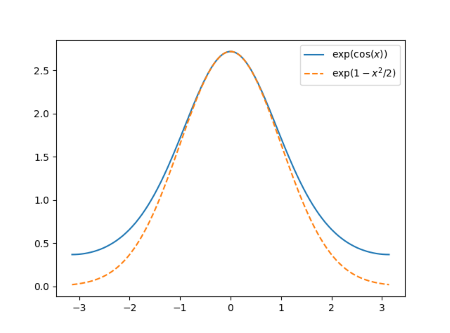Both functions are perfectly smooth. The function g is naturally periodic with period 2π. The function h could be modified to be a periodic function with the same period since h(-π) = h(π).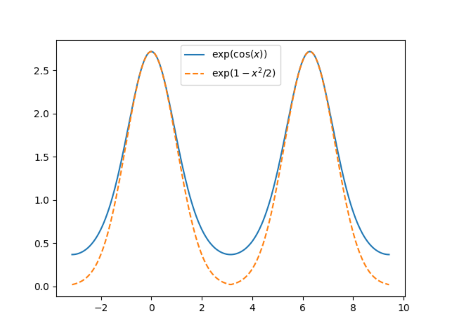But the periodic extension of h is not smooth. It’s continuous, but it has a kink at odd multiples of π. The derivative is not continuous at these points. Here’s a close-up to show the kink.Now suppose we want to integrate both functions from -π to π. Over that range both functions are smooth. But the behavior of h “off stage” effects the efficiency of the trapezoid rule. Making h periodic by pasting copies together that don’t match up smoothly does not make it act like a smooth periodic function as far as integration is concerned.

Here’s the error in the numerical integration using 2, 3, 4, …, 10 integration points.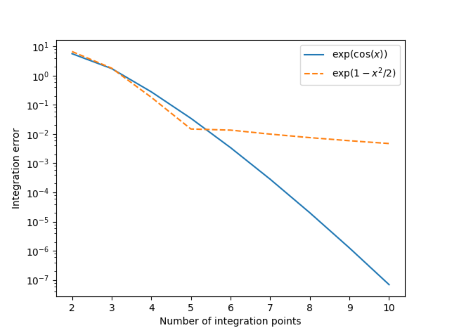The integration error for both functions decreases rapidly as we go from 2 to 5 integration points. And in fact the integration error for h is slightly less than that for g with 5 integration points. But the convergence for h practically stops at that point compared to g where the integration error decreases exponentially. Using only 10 integration points, the error has dropped to approximately 7×10-8 while the error for h is five orders of magnitude larger.

To read more along these lines, see J. A. C. Weideman’s article Numerical Integration of Periodic Functions: A Few Examples, The American Mathematical Monthly, Vol. 109, No. 1, pp. 21–36.